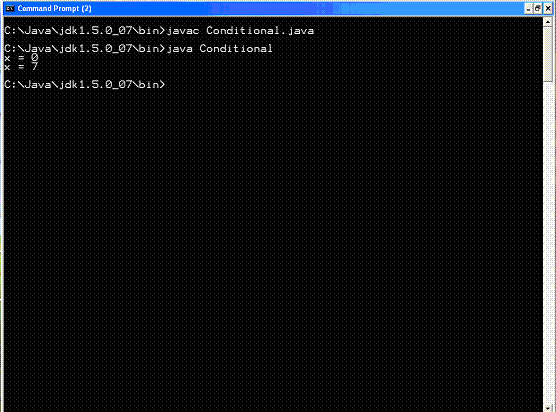## Core Java

Java Evolution
Overview of Java Language
Constant, Variable and Datatype
Operator and Expression
Decision making, Branching and Looping
Class Object and Method
Array and String
Inheritance
Package
Exception Handling
Applet
Managing Input/Output Files in Java
Examples of Core Java

# Operator and Expression

### Relational Floating-Point Operators

The relational floating-point operators compare two floating-point operands, leaving a boolean result.

Boolean Operators
Boolean operators act on boolean types and return a boolean result.

The boolean operators

 Description Operator Evaluation AND & Evaluation OR | Evaluation XOR ^ Logical AND && Logical OR || Negation ! Equal-to == Not-equal-to != Conditional ?:

The evaluation operators (&, |, and ^) evaluate both sides of an expression before determining the result.

The following code shows how the evaluation AND operator is necessary for the complete evaluation of an expression:

while ((++x < 10) && (++y < 15)) {
System.out.println(x);
System.out.println(y);

}

The three boolean operators--negation, equal-to, and not-equal-to (!, ==, and !=)
The negation operator toggles the value of a boolean from false to true or from true to false, depending on the original value.
The equal-to operator simply determines whether two boolean values are equal (both true or both false).

Similarly, the not-equal-to operator determines whether two boolean operands are unequal.

The conditional boolean operator (? :) is the most unique of the boolean operators This operator also is known as the ternary operator because it takes three items: a condition and two expressions.

The syntax for the conditional operator follows:

Condition ? Expression1 : Expression2

The Condition, which itself is a boolean, is first evaluated to determine whether it is true or false. If Condition evaluates to a true result, Expression1 is evaluated. If Condition ends up being false, Expression2 is evaluated.
 The Conditional class class Conditional { public static void main (String args[]) { int x = 0; boolean isEven = false; System.out.println("x = " + x); x = isEven ? 4 : 7; System.out.println("x = " + x); } } The results of the Conditional program follow: x = 0 x = 7 Output: